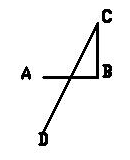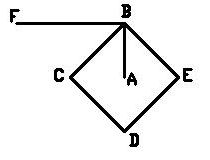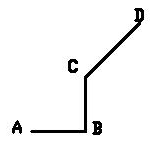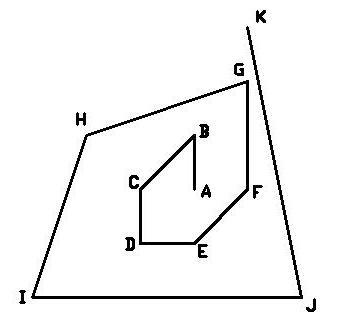Problem Statement

Given a set of points, choose the maximal number of them that can be used to form a spiral.

A spiral is formed as follows:

1. Start at point (0, 0).

2. Draw a line segment from the current point to one of the given points (x, y). (x, y) is now the new current point.

3. Repeat step 2 as many times as possible while obeying the following rules:

• The spiral must not intersect itself. This means that no two line segments in the spiral can have any common points (except the common endpoints of neighboring segments).
• Each point must not be used more than once.
• When going from each line segment to the next, you must turn counterclockwise and go forward. The angle you turn must be greater than or equal to 0, and strictly less than 180 degrees.
• The ray that originates from the second to last point and goes through the last point must not intersect any of the other line segments in the spiral.

These three spirals do not satisfy the first, second and third rules:These two do not satisfy the fourth rule:You are given a String[] points, each element of which represents a single point whose coordinates are given as "X Y" (quotes for clarity). Return the maximal number of points that can be used to form a single spiral.

Definition

 Class: SpiralConstruction Method: longestSpiral Parameters: String[] Returns: int Method signature: int longestSpiral(String[] points) (be sure your method is public)

Constraints

-points will contain between 1 and 15 elements, inclusive.
-Each element of points will be formatted as "X Y" (quotes for clarity), where X and Y are integers with no extra leading zeros.
-Each X and Y will be between -1000 and 1000, inclusive.
-All elements in points will be distinct.
-There will be no "0 0" in points.

Examples

0)

 {"0 1", "1 0"}
Returns: 2
 (0,0) -> (1,0) -> (0,1) is a valid spiral.
1)

 {"1 1", "2 2", "-1 -1"}
Returns: 2
 (0,0) -> (1,1) -> (2,2) is the longest valid spiral. Note that there is a turn by 0 degrees.
2)

 {"0 1", "1 1", "0 2"}
Returns: 2
 You can't form a valid spiral using all three points.
3)

 {"0 1", "-1 0", "0 -1", "1 2", "2 -2", "-2 1", "1 0", "-1 -1", "1 3", "-3 -2"}
Returns: 10Problem url:

http://www.topcoder.com/stat?c=problem_statement&pm=6649

Problem stats url:

http://www.topcoder.com/tc?module=ProblemDetail&rd=10791&pm=6649

Vedensky

Testers:

PabloGilberto , gawry , lovro , ivan_metelsky

Problem categories:

Dynamic Programming